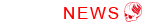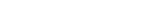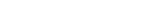Tips for Oversea Customers

How to calculate Final Reduction Ratio (2019-12-17)

This tip is for GALM users which how to calculate final reduction ratio.

First, you need to calculate primary(Spur Gear, Pinion Gear) and secondary(Diff Pulley, Center Pulley) reduction ratio. For each calculation formula are show on below.

Primary Reduction Ratio

 Spur Gear = Primary Reduction Ratio Pinion Gear

Secondary Reduction Ratio

 Diff Pulley = Secondary Reduction Ratio Center Pulley

Let's calculate how is the standard GALM primary and secondary reduction ratio. Here is standard GALM each gear and pulley.

Spur Gear : 92T

Pinion Gear : 23T

Diff Pulley : 39T

Center Pulley : 12T

Primary Reduction Ratio

 92T = 4.00 23T

Secondary Reduction Ratio

 39T = 3.25 12T

Now, you can see each reduction ratio. Then, next is calculation for final reduction ratio.

Primary Reduction Ratio × Secondary Reduction Ratio = Final Reduction Ratio

then this is going to be,

4.00 × 3.25 = 13.00

So, the final reduction ratio is 13.00.

Also, here is calculation when using Gear Drive Set.

Secondary Reduction Ratio for Gear Drive

 Diff Gear = Secondary Reduction Ratio Counter Gear

And here is standard gear drive set ratio (Diff Gear : 54T, Counter Gear 27T).

 54T = 2.00 27T

And the final reduction ratio is,

4.00 × 2.00 = 8.00

Calculation is pretty simple so please make use of your machine setting.

Copyright 2015 Weld Technique Factory Co. All Right Reserved.